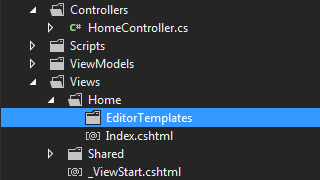When developing web applications , you may need to show radio button list in ASP.NET MVC  so that users can make a selection from the options(radio buttons) and submit the form.  We can do this easily with the help of MVC Model binding.

Let's create a quiz page where we are going to show some questions with it's answers to the user and user can select an answer from the available answer options, represented by radio buttons.

So first we will create some ViewModels for our view. ViewModel is nothing but a POCO class which we will use to transfer data between the action method and the view. So let's create 3 classes like this.

```public class Question
{
public int ID { set; get; }
public string QuestionText { set; get; }
public List<Answer> Answers { set; get; }
public string SelectedAnswer { set; get; }
public Question()
{
Answers = new List<Answer>();
}
}
public class Answer
{
public int ID { set; get; }
public string AnswerText { set; get; }
}
public class Evaluation
{
public List<Question> Questions { set; get; }
public Evaluation()
{
Questions = new List<Question>();
}
}```

Now,  in our GET action method for the view, we will create an object of our ViewModel (`Evaluation`) class and set the Questions and it's Answers properties and then send that to the view by passing it to the View method.

```public ActionResult Index()
{
var evalVM = new Evaluation();

//the below is hardcoded for DEMO. you may get the data from some
//other place and set the questions and answers

var q1 = new Question { ID = 1, QuestionText = "What is your favourite language" };
q1.Answers.Add(new Answer { ID = 12, AnswerText = "PHP" });
q1.Answers.Add(new Answer { ID = 13, AnswerText = "ASP.NET" });
q1.Answers.Add(new Answer { ID = 14, AnswerText = "Java" });
evalVM.Questions.Add(q1);

var q2 = new Question { ID = 2, QuestionText = "What is your favourite DB" };
q2.Answers.Add(new Answer { ID = 16, AnswerText = "SQL Server" });
q2.Answers.Add(new Answer { ID = 17, AnswerText = "MyQL" });
q2.Answers.Add(new Answer { ID = 18, AnswerText = "Oracle" });
evalVM.Questions.Add(q2);

return View(evalVM);
}```

Now our next step is to create the views to render the UI.  We are going to create an editor template to render the questions and it's answers. So Let's go to the `Views` folder and create a folder called EditorTemplates under the current controller folder (if you added the GET action method to `Home` controller, this will be `~/Views/Home`)After creating the folder, Add a new Editor template to that. Right click on the folder and select Add View option and give the name same as the type name (in this case` Question`).Now add the below code to the newly created editor template (Question.cshtml)

```@model ViewModels.Question
<div>
@Html.HiddenFor(x=>x.ID)
<h3> @Model.QuestionText </h3>
@foreach (var a in Model.Answers)
{
<p>
@Html.RadioButtonFor(b=>b.SelectedAnswer,a.ID)  @a.AnswerText
</p>
}
</div>```

What we are doing in the above code is, iterating through each Answers and showing a Radio button and the answer text. We are using the `RadioButtonFor` html helper method to render the radio button.

Now let's  go to our main view (index.cshtml) and write some code. Our main view will be strongly typed to our `Evaluation` viewmodel and we will use the ` EditorFor` html helper method to bring our editor template to the main view(index.cshtml).

```@model ViewModels.Evaluation
<h2>Quiz 24</h2>
@using (Html.BeginForm())
{
@Html.EditorFor(x=>x.Questions)
<input type="submit" />
}```

now run the app (hit F5) and you will see the screen with the questions and answers. each answer will be accompanied by a radio button so that user can select one.### Getting the selected radio button values on form post

To get the selected radio button values on form submit, we can check the properties of the posted model. MVC Model binding can bind the posted form data to an instance of our ViewModel. So let's add the below code to handle the POST action method.

```[HttpPost]
public ActionResult Index(Evaluation model)
{
if (ModelState.IsValid)
{
foreach (var q in model.Questions)
{
var qId = q.ID;
var selectedAnswer = q.SelectedAnswer;
// Save the data
}
return RedirectToAction("ThankYou"); //PRG Pattern
}
//to do : reload questions and answers
return View(model);
}```

Now if you run the program and use visual studio break points, you can see that the selected radio button values (AnswerID in our case) is available in the `SelectedAnswer` property of each question.Hope this helps. You can download the sample source code here. Do not forget to say "Hi" to me,  if this post was useful to you.